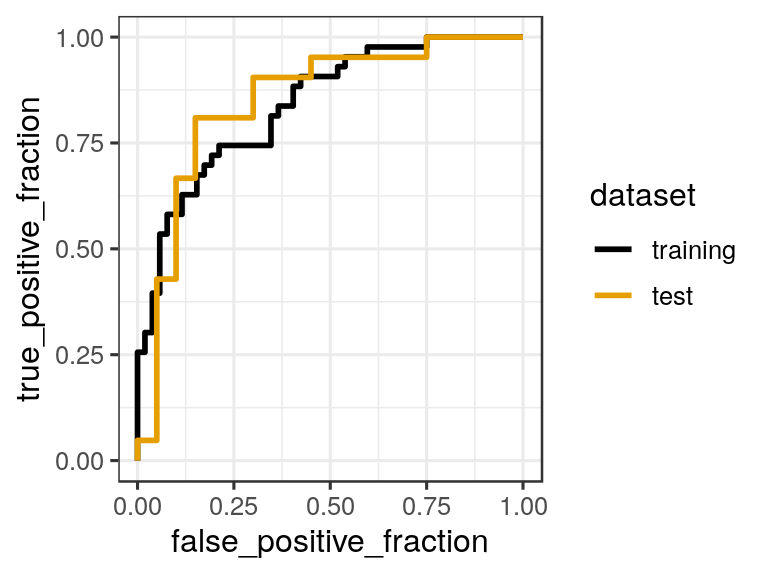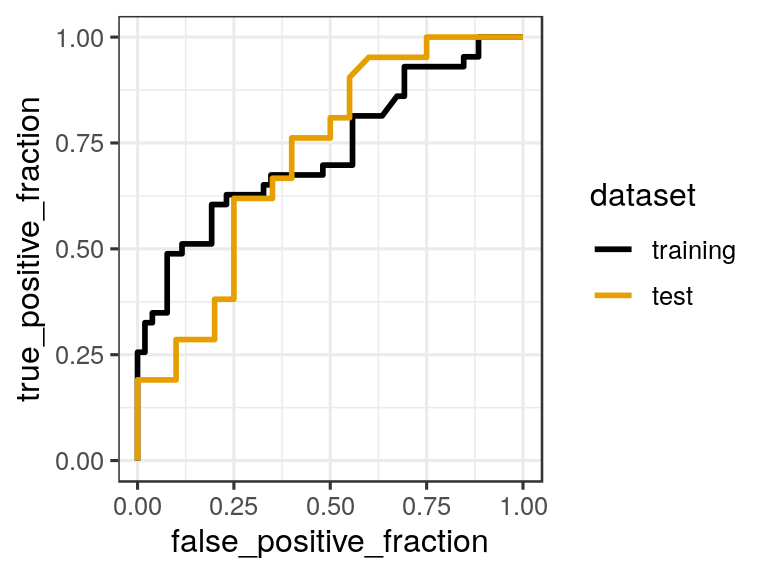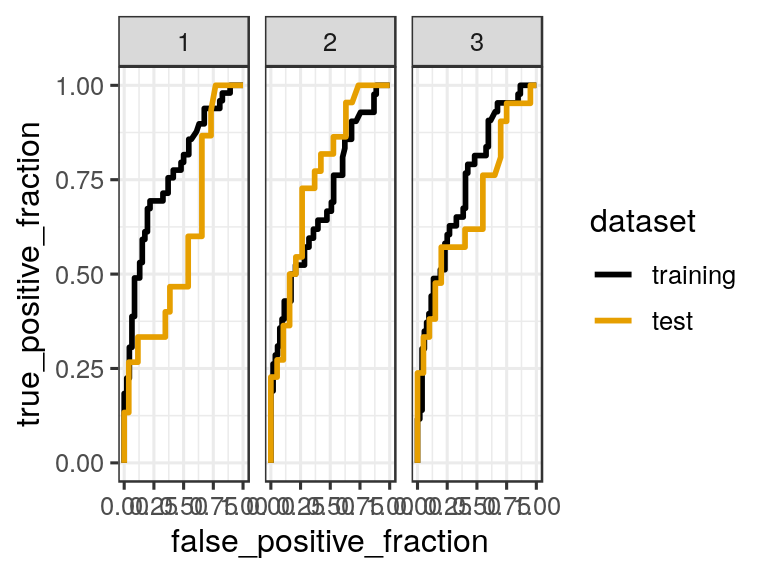## Lab Worksheet 7

In 1898, Hermon Bumpus, an American biologist working at Brown University, collected data on one of the first examples of natural selection directly observed in nature. Immediately following a bad winter storm, he collected 136 English house sparrows, Passer domesticus, and brought them indoors. Of these birds, 64 had died during the storm, but 72 recovered and survived. By comparing measurements of physical traits, Bumpus demonstrated physical differences between the dead and living birds. He interpreted this finding as evidence for natural selection as a result of this storm:

bumpus <- read.csv("http://wilkelab.org/classes/SDS348/data_sets/bumpus_full.csv")
head(bumpus)
##    Sex   Age Survival Length Wingspread Weight Skull_Length Humerus_Length
## 1 Male Adult    Alive    154        241   24.5         31.2           17.4
## 2 Male Adult    Alive    160        252   26.9         30.8           18.7
## 3 Male Adult    Alive    155        243   26.9         30.6           18.6
## 4 Male Adult    Alive    154        245   24.3         31.7           18.8
## 5 Male Adult    Alive    156        247   24.1         31.5           18.2
## 6 Male Adult    Alive    161        253   26.5         31.8           19.8
##   Femur_Length Tarsus_Length Sternum_Length Skull_Width
## 1         17.0          26.0           21.1        14.9
## 2         18.0          30.0           21.4        15.3
## 3         17.9          29.2           21.5        15.3
## 4         17.5          29.1           21.3        14.8
## 5         17.9          28.7           20.9        14.6
## 6         18.9          29.1           22.7        15.4

The data set has three categorical variables (Sex with levels Male and Female; Age with levels Adult and Young; and Survival, with levels Alive and Dead) and nine numerical variables that hold various aspects of the birds’ anatomy, such as wingspread, weight, etc.

Split the bumpus data set into a random training and test set. Use 70% of the data as a training set.

set.seed(13)  # set the seed to make your partition reproductible

train_fraction <- 0.7 # fraction of data for training purposes
train_size <- floor(train_fraction * nrow(bumpus)) # number of observations in training set
train_indices <- sample(1:nrow(bumpus), size = train_size)

train_data <- bumpus[train_indices, ] # get training data
test_data <- bumpus[-train_indices, ] # get test data

Fit a logistic regression model on the training data set, then predict the survival on the test data set, and plot the resulting ROC curves.

# model to use:
# Survival ~ Sex + Length + Weight + Humerus_Length + Sternum_Length

# make training model
glm_out_train <- glm(Survival ~ Sex + Length + Weight + Humerus_Length + Sternum_Length,
data=train_data,
family=binomial)

# results data frame for training data
df_train <- data.frame(predictor = predict(glm_out_train, train_data),
known_truth = train_data$Survival, dataset = "training") # results data frame for test data df_test <- data.frame(predictor = predict(glm_out_train, test_data), known_truth = test_data$Survival,
dataset = "test")

df_combined <- rbind(df_train, df_test)

ggplot(df_combined, aes(d = known_truth, m = predictor, color = dataset)) +
geom_roc(n.cuts = 0) +
scale_color_colorblind()
## Warning in verify_d(data$d): D not labeled 0/1, assuming Alive = 0 and Dead ## = 1!# 2. Area under the ROC curves Calculate the area under the training and test curve for this following model. # model to use: # Survival ~ Weight + Humerus_Length set.seed(13) # set the seed to make your partition reproductible train_fraction <- 0.7 # fraction of data for training purposes train_size <- floor(train_fraction * nrow(bumpus)) # number of observations in training set train_indices <- sample(1:nrow(bumpus), size = train_size) train_data <- bumpus[train_indices, ] # get training data test_data <- bumpus[-train_indices, ] # get test data glm_out_train <- glm(Survival ~ Weight + Humerus_Length, data=train_data, family=binomial) # results data frame for training data df_train <- data.frame(predictor = predict(glm_out_train, train_data), known_truth = train_data$Survival,
dataset = "training")

# results data frame for test data
df_test <- data.frame(predictor = predict(glm_out_train, test_data),
known_truth = test_data$Survival, dataset = "test") df_combined <- rbind(df_train, df_test) p <- ggplot(df_combined, aes(d = known_truth, m = predictor, color = dataset)) + geom_roc(n.cuts = 0) + scale_color_colorblind() p ## Warning in verify_d(data$d): D not labeled 0/1, assuming Alive = 0 and Dead
## = 1!calc_auc(p)
## Warning in verify_d(data$d): D not labeled 0/1, assuming Alive = 0 and Dead ## = 1! ## PANEL group AUC ## 1 1 1 0.7298748 ## 2 1 2 0.7178571 model_identifiers <- unique(df_combined$dataset)
model_identifiers_info <- data.frame(model_identifiers,
group = order(model_identifiers))

left_join(model_identifiers_info, calc_auc(p)) %>%
select(-group, -PANEL) %>%
arrange(desc(AUC))
## Warning in verify_d(data$d): D not labeled 0/1, assuming Alive = 0 and Dead ## = 1! ## Joining, by = "group" ## model_identifiers AUC ## 1 training 0.7298748 ## 2 test 0.7178571 # 3. What happens if we don’t use set.seed() to create reproducibility in the way we partition the dataset? ######## random partition 1 train_fraction1 <- 0.7 # fraction of data for training purposes train_size1 <- floor(train_fraction1 * nrow(bumpus)) # number of observations in training set train_indices1 <- sample(1:nrow(bumpus), size = train_size1) train_data1 <- bumpus[train_indices1, ] # get training data test_data1 <- bumpus[-train_indices1, ] # get test data glm_out_train1 <- glm(Survival ~ Weight + Humerus_Length, data=train_data1, family=binomial) # results data frame for training data df_train1 <- data.frame(predictor = predict(glm_out_train1, train_data1), known_truth = train_data1$Survival,
dataset = "training")

# results data frame for test data
df_test1 <- data.frame(predictor = predict(glm_out_train1, test_data1),
known_truth = test_data1$Survival, dataset = "test") df_combined1 <- rbind(df_train1, df_test1) %>% mutate(random_partition = "1") ######## random partition 2 train_fraction2 <- 0.7 # fraction of data for training purposes train_size2 <- floor(train_fraction2 * nrow(bumpus)) # number of observations in training set train_indices2 <- sample(1:nrow(bumpus), size = train_size2) train_data2 <- bumpus[train_indices2, ] # get training data test_data2 <- bumpus[-train_indices2, ] # get test data glm_out_train2 <- glm(Survival ~ Weight + Humerus_Length, data=train_data2, family=binomial) # results data frame for training data df_train2 <- data.frame(predictor = predict(glm_out_train2, train_data2), known_truth = train_data2$Survival,
dataset = "training")

# results data frame for test data
df_test2 <- data.frame(predictor = predict(glm_out_train2, test_data2),
known_truth = test_data2$Survival, dataset = "test") df_combined2 <- rbind(df_train2, df_test2) %>% mutate(random_partition = "2") ######## random partition 3 train_fraction3 <- 0.7 # fraction of data for training purposes train_size3 <- floor(train_fraction3 * nrow(bumpus)) # number of observations in training set train_indices3 <- sample(1:nrow(bumpus), size = train_size3) train_data3 <- bumpus[train_indices3, ] # get training data test_data3 <- bumpus[-train_indices3, ] # get test data glm_out_train3 <- glm(Survival ~ Weight + Humerus_Length, data=train_data3, family=binomial) # results data frame for training data df_train3 <- data.frame(predictor = predict(glm_out_train3, train_data3), known_truth = train_data3$Survival,
dataset = "training")

# results data frame for test data
df_test3 <- data.frame(predictor = predict(glm_out_train3, test_data3),
known_truth = test_data3$Survival, dataset = "test") df_combined3 <- rbind(df_train3, df_test3) %>% mutate(random_partition = "3") ######## plot all 3 df_all <- rbind(df_combined1, df_combined2, df_combined3) p_all <- ggplot(df_all, aes(d = known_truth, m = predictor, color = dataset)) + geom_roc(n.cuts = 0) + scale_color_colorblind() + facet_wrap(~random_partition) p_all ## Warning in verify_d(data$d): D not labeled 0/1, assuming Alive = 0 and Dead
## = 1!Randomly selecting a subset of data for the training and testing data creates significant inconsistency in the performance of the model in classifying the test data; the performance of the model relative to the training data stays relatively consistent.